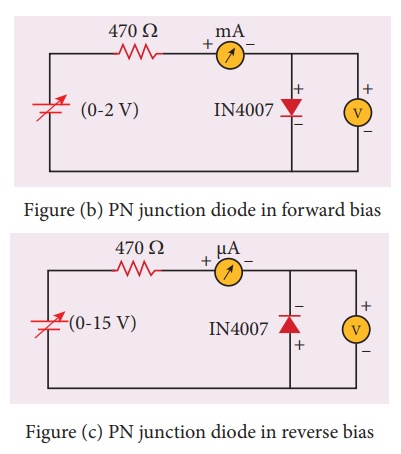Home | | Physics 12th Std | Voltage-Current Characteristics of a PN Junction Diode

# Voltage-Current Characteristics of a PN Junction Diode

To draw the voltage-current (V- I) characteristics of the PN junction diode and to determine its knee voltage and forward resistance.

VOLTAGE-CURRENT CHARACTERISTICS OF A PN JUNCTION DIODE

### AIM

To draw the voltage-current (V- I) characteristics of the PN junction diode and to determine its knee voltage and forward resistance.

### APPARATUS REQUIRED

PN junction diode (IN4007), variable DC power supply, milli-ammeter, micro-ammeter, voltmeter, resistance and connecting wires.

### FORMULAwhere,

RF ŌåÆ Forward resistance of the diode (╬®)

ŌłåVF ŌåÆ The change in forward voltage (volt)

ŌłåIF  ŌåÆ The change in forward current (mA)

### CIRCUIT DIAGRAMFigure (a) PN junction diode and its symbol (Silver ring denotes the negative terminal of the diode)

Figure (b) PN junction diode in forward biasFigure (c) PN junction diode in reverse bias

### Precaution

Care should be taken to connect the terminals of ammeter, voltmeter, dc power supply and the PN junction diode with right polarity.

### i) Forward bias characteristics

┬Ę        In the forward bias, the P- region of the diode is connected to the positive terminal and N-region to the negative terminal of the DC power supply.

┬Ę        The connections are given as per the circuit diagram.

┬Ę        The voltage across the diode can be varied with the help of the variable DC power supply.

┬Ę        The forward voltage (VF) across the diode is increased from 0.1ŌĆåV in steps of 0.1ŌĆåV up to 0.8|V and the forward current (IF) through the diode is noted from the milli-ammeter. The readings are tabulated.

┬Ę        The forward voltage VF and the forward current IF are taken as positive.

┬Ę        A graph is drawn taking the forward voltage (VF) along the x-axis and the forward current (IF) along the y-axis.

┬Ę        The voltage corresponding to the dotted line in the forward characteristics gives the knee voltage or threshold voltage or turn-on voltage of the diode.

┬Ę        The slope in the linear portion of the forward characteristics is calculated. The reciprocal of the slope gives the forward resistance of the diode.

### ii) Reverse bias characteristics

┬Ę        In the reverse bias, the polarity of the DC power supply is reversed so that the P- region of the diode is connected to the negative terminal and N-region to the positive terminal of the DC power supply

┬Ę        The connections are made as given in the circuit diagram.

┬Ę        The voltage across the diode can be varied with the help of the variable DC power supply.

┬Ę        The reverse voltage (VR) across the diode is increased from 1ŌĆåV in steps of 1ŌĆåV up to 5ŌĆåV and the reverse current (IR) through the diode is noted from the micro-ammeter. The readings are tabulated.

┬Ę        The reverse voltage VR and reverse current IR are taken as negative.

┬Ę        A graph is drawn taking the reverse bias voltage (VR) along negative x-axis and the reverse bias current (IR) along negative y-axis.### OBSERVATION### CALCULATION

(i) Forward resistance RF =

(ii) knee voltage =

### RESULT

The V-I characteristics of the PN junction diode are studied.

i) Knee voltage of the PN junction diode =ŌĆ”ŌĆ”ŌĆ”ŌĆ”ŌĆ”ŌĆ”V

ii) Forward resistance of the diode =ŌĆ”ŌĆ”ŌĆ”ŌĆ”ŌĆ”ŌĆ”Ōä”

### Practical Tips

┬Ę        The DC power supply voltage should be increased only up to the specified range in the for-ward (0 ŌĆō 2V) and reverse (0 ŌĆō 15V) directions. Forward bias offers very low resistance and hence an external resistance of 470╬® is connected as a safety measure.

┬Ę        The voltage applied beyond this limit may damage the resistance or the diode.

┬Ę        In the forward bias, the current flow will be almost zero till it crosses the junction potential or knee voltage (approximately 0.7ŌĆåV). Once knee voltage is crossed, the current increases with the applied voltage.

┬Ę        The diode voltage in the forward direction should be increased in steps of 0.1ŌĆåV to a maximum of 0.8ŌĆåV after the threshold voltage to calculate the forward resistance.

┬Ę        The diode voltage in the reverse direction is increased in steps of 1ŌĆåV to a maximum of 5ŌĆåV. The current must be measured using micro-ammeter as the strength of current in the reverse direction is very less. This is due to the flow of the minority charge carriers called the leakage current.

Tags : Physics Practical Experiment , 12th Physics : Practical
Study Material, Lecturing Notes, Assignment, Reference, Wiki description explanation, brief detail
12th Physics : Practical : Voltage-Current Characteristics of a PN Junction Diode | Physics Practical Experiment